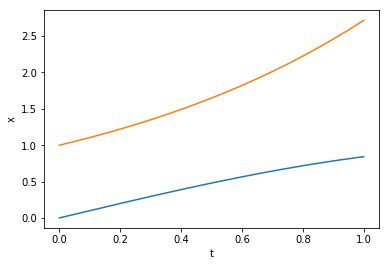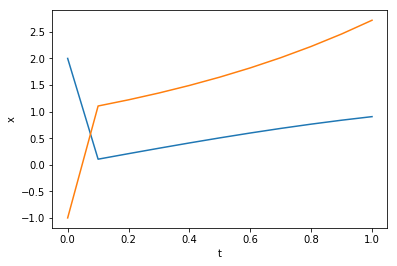## Implicit Euler for DAEs¶

For a general DAE of type $$F(t, x, \dot x)=0$$ the Implicit Euler scheme that advances the current approximation $x_k$ at time step $t_k$ to the approximation $x_{k+1}$ at time step $t_{k+1}$ is defined through $$F(t, x_{k+1}, \frac{x_{k+1}-x_k}{h})=0,$$ where $h$ is the time-step length. If $F$ is a nonlinear function, then a nonlinear solver is needed to solve for $x_{k+1}$.

In :
import numpy as np
import matplotlib.pyplot as plt
from scipy.optimize import fsolve

def impliciteuler(F=None, inival=None, interval=[0, 1], Nts=100,
dFdx=None, dFdxdot=None):
"""
Parameters:
---
F: callable
the problem in the form F(t,x,x')
Nts: integer
Number of time steps, defaults to 100.
"""
t0, te = interval, interval
h = 1./Nts*(te-t0)
N = inival.size

sollist = [inival.reshape((1, N))]
tlist = [t0]

xk = inival
for k in range(1, Nts+1):  # python starts counting with zero...
tkk = t0 + k*h

def impeuler_increment(xkkn):
""" the implicit Euler update for a general F """
return F(tkk,xkkn,1./h*(xkkn-xk)).flatten()

xkk, _, flag, msg = fsolve(func=impeuler_increment, x0=xk, full_output=True)
# call a nonlinear solver for the system f(x)=0
if not flag == 1:
print('Caution at t={0}: '.format(tkk) + msg)
sollist.append(xkk.reshape((1, N)))
tlist.append(tkk)
xk = xkk

sol = np.vstack(sollist)
plt.plot(tlist, sol)
plt.xlabel('t'), plt.ylabel('x')
plt.show()

return sol


## Linear DAEs with time-varying coefficients¶

Let $F(t, x, \dot x)= E(t)\dot x - A(t)x - f(t)$.

### Problem (a)¶

Consider \begin{equation*} E(t) = \begin{bmatrix} -t &t^2 \\ -1 &t \end{bmatrix}, \quad A(t) = \begin{bmatrix} -1 &0 \\ 0 &-1 \end{bmatrix}, \quad f(t) = \begin{bmatrix} 0 \\ 0 \end{bmatrix}. \end{equation*} with the initial value $x_0=[0,1]^T$ on the interval $\mathbb I = [0,10]$.

The problem has infinitely many solutions, namely $x(t) = c(t)\begin{bmatrix} t \\ 1 \end{bmatrix}$ for any smooth scalar function $t$. The Implicit Euler nevertheless returns a solution (without telling you that there are there are many!!!)

### Problem (b)¶

Consider \begin{equation*} E(t) = \begin{bmatrix} 0 & 0 \\ 1 & -1 \end{bmatrix}, \quad A(t) = \begin{bmatrix} -1 &t \\ 0 & 0 \end{bmatrix}, \quad f(t) = \begin{bmatrix} \sin(t) \\ \cos(t) \end{bmatrix}. \end{equation*}
with the initial value $x_0=[1,1]^T$ on the interval $\mathbb I = [0,10]$. This problem has the unique solution $x(t) = \begin{bmatrix} \sin(t) \\ 0 \end{bmatrix}$. Thus, the provided initial value ist not consistent. Anyways, also with the consistent solution the Implicit Euler does not give a (reasonable) solution but issues a warning that at some point the equation for $x_{k+1}$ cannot be solved satisfactory. In fact, at $t=1$, the matrix $E(t)-hA(t)$, which is inverted when solving for $x_{k+1}$ is singular (for any $h$). This is an example, where there is a unique solution, but the Implicit Euler fails.

### Problem (c)¶

Consider \begin{equation*} E(t) = \begin{bmatrix} 1 & 1 \\ 0 & 0 \end{bmatrix}, \quad A(t) = \begin{bmatrix} 0 &0 \\ 0 &-1 \end{bmatrix}, \quad f(t) = \begin{bmatrix} e^t+\cos(t) \\ e^t \end{bmatrix} \end{equation*} with $x_0=[0,1]^T$, $\mathbb I = [0,1]$. This problem has the unique solution $x(t) = \begin{bmatrix} \sin(t) \\ \exp(t) \end{bmatrix}$ and the Implicit Euler works just fine. Observe, that an inconsistent initial value (here: $x_0=[2,-1]^T$) is "corrected" in the first time-step.

In :
## Problem (a)

def E(t):
return np.array([[-t, t*t], [-1, t]])
def A(t):
return np.array([[-1, 0], [0, -1]])
def f(t):
return np.array([0, 0])

def F(t, x, xdot):
return E(t).dot(xdot) - A(t).dot(x) - f(t)

inival = np.array([0, 1])
interval = [0, 10]

sol = impliciteuler(F=F, inival=inival, interval=interval, Nts=500)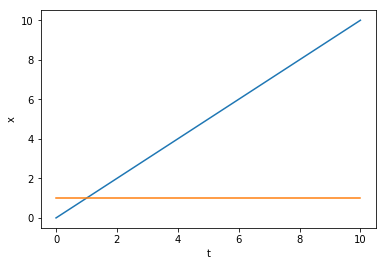In :
## Problem (b)

def E(t):
return np.array([[0, 0], [1, -1]])
def A(t):
return np.array([[-1, t], [0, 0]])
def f(t):
return np.array([np.sin(t), np.cos(t)])

def F(t, x, xdot):
return E(t).dot(xdot) - A(t).dot(x) - f(t)

interval = [0, 2]

inival = np.array([1, 1])
sol = impliciteuler(F=F, inival=inival, interval=interval, Nts=100)

inival = np.array([0, 0])
sol = impliciteuler(F=F, inival=inival, interval=interval, Nts=100)

Caution at t=1.0: The iteration is not making good progress, as measured by the
improvement from the last ten iterations.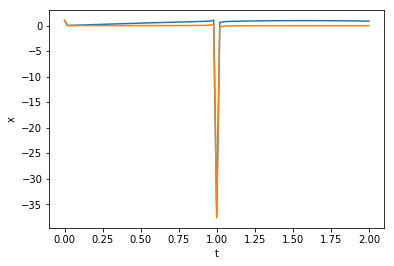Caution at t=1.0: The iteration is not making good progress, as measured by the
improvement from the last ten iterations.
Caution at t=1.02: The iteration is not making good progress, as measured by the
improvement from the last ten iterations.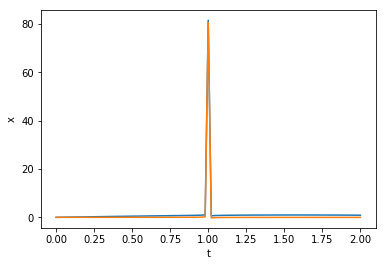In :
## Problem (c)

def E(t):
return np.array([[1, 1], [0, 0]])
def A(t):
return np.array([[0, 0], [0, -1]])
def f(t):
return np.array([np.exp(t)+np.cos(t), np.exp(t)])

def F(t, x, xdot):
return E(t).dot(xdot) - A(t).dot(x) - f(t)

interval = [0, 1]

inival = np.array([0, 1])
sol = impliciteuler(F=F, inival=inival, interval=interval, Nts=1000)

inival = np.array([2, -1])
sol = impliciteuler(F=F, inival=inival, interval=interval, Nts=10)